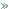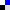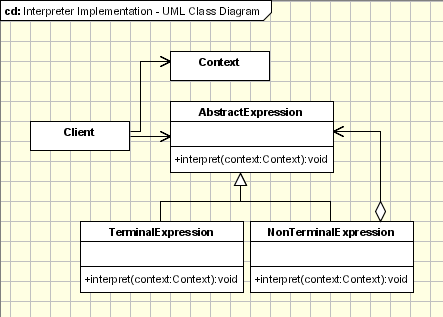HomeBehavioral PatternsInterpreterBookmark this on Delicious

Interpreter

Motivation

The Interpreter is one of the Design Patterns published in the GoF which is not really used. Ussualy the Interpreter Pattern is described in terms of formal grammars, like it was described in the original form in the GoF but the area where this design pattern can be applied can be extended.

Intent

- Given a language, define a representation for its grammar along with an interpreter that uses the representation to interpret sentences in the language.
- Map a domain to a language, the language to a grammar, and the grammar to a hierarchical object-oriented design

Implementation

The implementation of the Interpreter pattern is just the use of the composite pattern applied to represent a grammar. The Interpreter defines the behaviour while the composite defines only the structure.Applicability & Examples

The Template Method pattern should be used:
- The Interpreter pattern is used exaustively in defining grammars, tokenize input and store it.
- A specific area where Interpreter can be used are the rules engines.
- The Interpreter pattern can be used to add functionality to the composite pattern.

Example 1 - Roman Numerals Convertor

The classical example fot the interpreter pattern is the one of interpreting the roman numerals. The expresion to be interpreted is a string which is put in the context. The context consists of the remaining unparsed Roman Numeral string and of the result of the numerral that are already parsed. The context is passed to one of four sub-interpreters based on the type of interpreting(Thousand, Hundred, Ten, One). This example it's using only TerminalExpressions.
The following participant classes are involved in this example: Context - keeps the current string that has to be parsed and the decimal that contains the conversion already done. Initially the context keeps the full string that has to be converted and 0 for the output decimal.

Expression - Consists of the interpret method which receives the context. Based on the current object it uses specific values for Thousand, Hundred, Ten, One and a specific multiplier.

ThousandExpression, HundredExpression, TenExpression, OneExpression (TerminalExpression) - Those classes are usued to define each specific expression. Ussualy, the TerminalExpression classes implement the interpret method. In our case the method is already defined in the base Expression class and each TerminalExpression class defines its behaviour by implmenting the abstract methods: one, four(), five(), nine(), multiplier(). It is a template method pattern.

Main(Client) - In our litle example this class is responsible to build the syntax tree representing a specific sentence in the language defined by the grammar. After the syntax tree is build the main method is invoking the interpret method.
 public class Context { private String input; private int output; public Context(String input) { this.input = input; } public String getInput() { return input; } public void setInput(String input) { this.input = input; } public int getOutput() { return output; } public void setOutput(int output) { this.output = output; } }public abstract class Expression { public void interpret(Context context) { if (context.getInput().length() == 0) return; if (context.getInput().startsWith(nine())) { context.setOutput(context.getOutput() + (9 * multiplier())); context.setInput(context.getInput().substring(2)); } else if (context.getInput().startsWith(four())) { context.setOutput(context.getOutput() + (4 * multiplier())); context.setInput(context.getInput().substring(2)); } else if (context.getInput().startsWith(five())) { context.setOutput(context.getOutput() + (5 * multiplier())); context.setInput( context.getInput().substring(1)); } while (context.getInput().startsWith(one())) { context.setOutput(context.getOutput() + (1 * multiplier())); context.setInput(context.getInput().substring(1)); } } public abstract String one(); public abstract String four(); public abstract String five(); public abstract String nine(); public abstract int multiplier(); }public class ThousandExpression extends Expression{ public String one() { return "M"; } public String four(){ return " "; } public String five(){ return " "; } public String nine(){ return " "; } public int multiplier() { return 1000; }}public class HundredExpression extends Expression{ public String one() { return "C"; } public String four(){ return "CD"; } public String five(){ return "D"; } public String nine(){ return "CM"; } public int multiplier() { return 100; }}public class TenExpression extends Expression{ public String one() { return "X"; } public String four(){ return "XL"; } public String five(){ return "L"; } public String nine(){ return "XC"; } public int multiplier() { return 10; }}public class OneExpression extends Expression{ public String one() { return "I"; } public String four(){ return "IV"; } public String five(){ return "V"; } public String nine(){ return "IX"; } public int multiplier() { return 1; }}public class MainInterpreter { /** * @param args */ public static void main(String[] args) { String roman = "MCMXXVIII"; Context context = new Context(roman); // Build the 'parse tree' ArrayList tree = new ArrayList(); tree.add(new ThousandExpression()); tree.add(new HundredExpression()); tree.add(new TenExpression()); tree.add(new OneExpression()); // Interpret for (Iterator it = tree.iterator(); it.hasNext();) { Expression exp = (Expression)it.next(); exp.interpret(context); } System.out.println(roman + " = " + Integer.toString(context.getOutput())); }}

Example 2 - Rule Validator

This example can be used to validate some conditions. Each expression is interpreted and the output for each expression is a boolean value. The following classes and entities are involved:

String(Context) - The String is used as a context. The string that has to be interpreted is parsed.

Expression - An abstract class with an abstract method called interpret.

TerminalExpression - This is the terminal expression. Only one terminal expression class is defined in our example and it returns true if a token was found there.

AlternationExpression, SequenceExpression(NonTerminalExpressions) - Implements logical operators(OR, AND) between 2 terminal or non terminal expressions.

Main(Client) - builds the abstract tree and call the interpret method of the Interpreter tree.

 public abstract class Expression { abstract public boolean interpret(String str); }public class TerminalExpression extends Expression { private String literal = null; public TerminalExpression(String str) { literal = str; } public boolean interpret(String str) { StringTokenizer st = new StringTokenizer(str); while (st.hasMoreTokens()) { String test = st.nextToken(); if (test.equals(literal)) { return true; } } return false; }}public class OrExpression extends Expression{ private Expression expression1 = null; private Expression expression2 = null; public OrExpression(Expression expression1, Expression expression2) { this.expression1 = expression1; this.expression2 = expression2; } public boolean interpret(String str) { return expression1.interpret(str) || expression2.interpret(str); } }public class AndExpression extends Expression{ private Expression expression1 = null; private Expression expression2 = null; public AndExpression(Expression expression1, Expression expression2) { this.expression1 = expression1; this.expression2 = expression2; } public boolean interpret(String str) { return expression1.interpret(str) && expression2.interpret(str); } }public class Main { /** * this method builds the interpreter tree * It defines the rule "Owen and (John or (Henry or Mary))" * @return */ static Expression buildInterpreterTree() { // Literal Expression terminal1 = new TerminalExpression("John"); Expression terminal2 = new TerminalExpression("Henry"); Expression terminal3 = new TerminalExpression("Mary"); Expression terminal4 = new TerminalExpression("Owen"); // Henry or Mary Expression alternation1 = new OrExpression(terminal2, terminal3); // John or (Henry or Mary) Expression alternation2 = new OrExpression(terminal1, alternation1); // Owen and (John or (Henry or Mary)) return new AndExpression(terminal4, alternation2); } /** * main method - build the interpreter * and then interpret a specific sequence * @param args */ public static void main(String[] args) { String context = "Mary Owen"; Expression define = buildInterpreterTree(); System.out.println(context + " is " + define.interpret(context)); }}

Conclusion

The Interpreter pattern has a limited area where it can be applied. We can dsicuss the Interpreter pattern only in terms of formal grammars but in this area there are better solutions and this is the reason why this pattern is not so frequenlty used. This pattern can be applied for parssing light expressions defined in simple grammars and sometimes in simple rule engines.